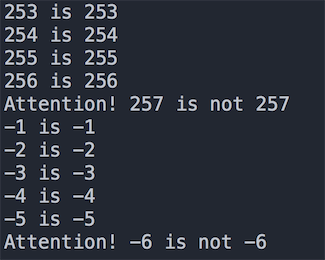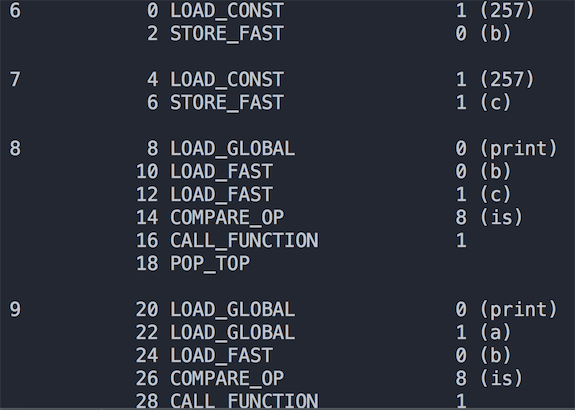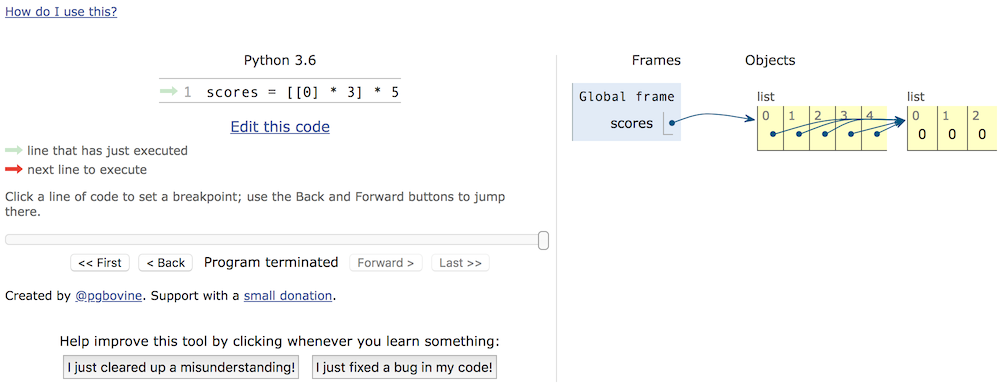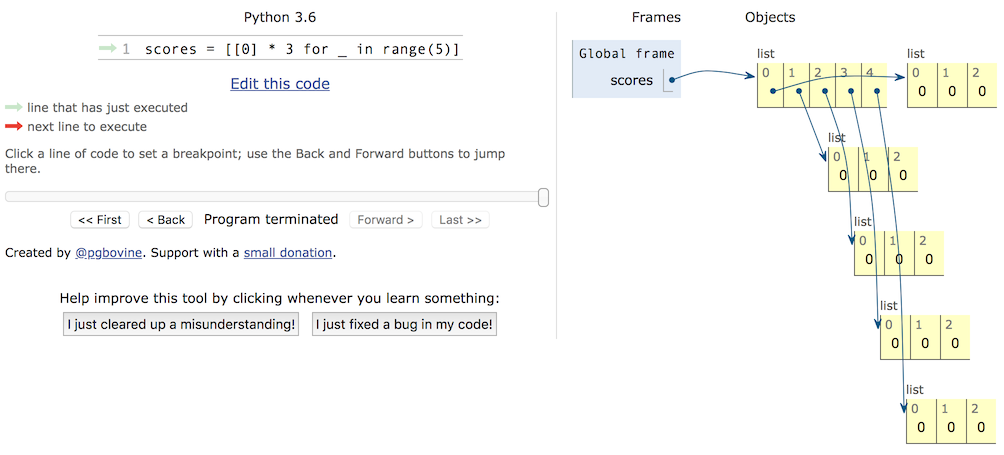# Python开发系列课程(10) - 那些年我们踩过的那些坑(上)

## 那些年我们踩过的那些坑### 坑01 - 整数比较的坑

• is比较的是两个整数对象的id值是否相等，也就是比较两个引用是否代表了内存中同一个地址。
• ==比较的是两个整数对象的内容是否相等，使用==时其实是调用了对象的__eq__()方法。

def main():
x = y = -1
while True:
x += 1
y += 1
if x is y:
print('%d is %d' % (x, y))
else:
print('Attention! %d is not %d' % (x, y))
break

x = y = 0
while True:
x -= 1
y -= 1
if x is y:
print('%d is %d' % (x, y))
else:
print('Attention! %d is not %d' % (x, y))
break

if __name__ == '__main__':
main()import dis
a = 257

def main():
b = 257  # 第6行
c = 257  # 第7行
print(b is c)  # True
print(a is b)  # False
print(a is c)  # False

if __name__ == "__main__":
main()


if __name__ == "__main__":
main()
dis.dis(main)### 坑02 - 嵌套列表的坑

Python中有一种内置的数据类型叫列表，它是一种容器，可以用来承载其他的对象（准确的说是其他对象的引用），列表中的对象可以称为列表的元素，很明显我们可以把列表作为列表中的元素，这就是所谓的嵌套列表。嵌套列表可以模拟出现实中的表格、矩阵、2D游戏的地图（如植物大战僵尸的花园）、棋盘（如国际象棋、黑白棋）等。但是在使用嵌套的列表时要小心，否则很可能遭遇非常尴尬的情况，下面是一个小例子。

def main():
names = ['关羽', '张飞', '赵云', '马超', '黄忠']
subjs = ['语文', '数学', '英语']
scores = [ * 3] * 5
for row, name in enumerate(names):
print('请输入%s的成绩' % name)
for col, subj in enumerate(subjs):
scores[row][col] = float(input(subj + ': '))
print(scores)

if __name__ == '__main__':
main()


a = object()
b = ['apple', 'pitaya', 'grape']

def main():
names = ['关羽', '张飞', '赵云', '马超', '黄忠']
subjs = ['语文', '数学', '英语']
scores = [[]] * 5
for row, name in enumerate(names):
print('请输入%s的成绩' % name)
scores[row] =  * 3
for col, subj in enumerate(subjs):
scores[row][col] = float(input(subj + ': '))
print(scores)

if __name__ == '__main__':
main()


def main():
names = ['关羽', '张飞', '赵云', '马超', '黄忠']
subjs = ['语文', '数学', '英语']
scores = [ * 3 for _ in range(5)]
for row, name in enumerate(names):
print('请输入%s的成绩' % name)
scores[row] =  * 3
for col, subj in enumerate(subjs):
scores[row][col] = float(input(subj + ': '))
print(scores)

if __name__ == '__main__':
main()### 坑03 - 访问修饰符的坑

class Student(object):

def __init__(self, name, age):
self.__name = name
self.__age = age

def __str__(self):
return self.__name + ': ' + str(self.__age)

def main():
stu = Student('骆昊', 38)
# 'Student' object has no attribute '__name'
# print(stu.__name)
# 用下面的方式照样可以访问类中的私有成员
print(stu._Student__name)
print(stu._Student__age)

if __name__ == '__main__':
main()


Python为什么要做出这样的设定呢？用一句广为流传的格言来解释这个问题：“We are all consenting adults here”（我们都是成年人）。这句话表达了很多Python程序员的一个共同观点，那就是开放比封闭要好，我们应该自己对自己的行为负责而不是从语言层面来限制对数据或方法的访问。

©️2019 CSDN 皮肤主题: 编程工作室 设计师: CSDN官方博客